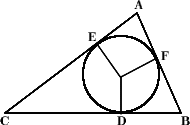#### You may also like### Degree Ceremony

What does Pythagoras' Theorem tell you about these angles: 90Â°, (45+x)Â° and (45-x)Â° in a triangle?### Logosquares

Ten squares form regular rings either with adjacent or opposite vertices touching. Calculate the inner and outer radii of the rings that surround the squares.### Ball Bearings

If a is the radius of the axle, b the radius of each ball-bearing, and c the radius of the hub, why does the number of ball bearings n determine the ratio c/a? Find a formula for c/a in terms of n.

# So Big

##### Age 16 to 18Challenge LevelA circle of radius $r$ is drawn inside a triangle so that it just touches each of the three sides as shown in the diagram. The three corners and points where the circle touches have been labelled $A$ to $F$. One side of the triangle is divided into segments of length $a$ and $b$ by the inscribed circle. However, we are not told which of the three sides is divided in this way. From this information we can find an expression for the area of the triangle. Prove that the area of the triangle is: $$\frac{abr(a+b)}{ab-r^2}.$$